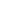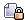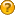anythingDid you miss your activation email?

###Author Topic: How do I create my own airport outline files?  (Read 10264 times)

0 Members and 1 Guest are viewing this topic.

#### AirNav Team

• AirNav Systems
• Jr. Member
•• Posts: 57##### How do I create my own airport outline files?
« on: March 19, 2008, 08:19:40 PM »
Here is an example outline file of Hamburg Airport created using Jordan's Outline Marker which can be found here:

http://www.acme.com/jef/outlines/

It's a point and click exercise which produces the outline file as shown below.  This is then copied into Windows Notepad and saved as an .out file such as Hamburg.out and placed in the Outlines directory (not the Outdirectory).  Scroll down to see a full description below the example outline file.

You may have to restart RadarBox and zoom in a bit to see the outline and make sure that your Airport colour in preferences isn't the same as the background colour.  Also, make sure you have Outlines selected in the Map menu.

You can copy the outline file below and past it to Notepad to see how it works.{Hamburg EDDH}
\$TYPE=2
53.65420+9.97486
53.63319+9.99310
53.61843+9.96340
53.61797+9.96400
53.61723+9.96387
53.61680+9.96417
53.61657+9.96490
53.61670+9.96572
53.61692+9.96623
53.62196+9.97657
53.62189+9.97709
53.62171+9.97735
53.62120+9.97803
53.62102+9.97799
53.62049+9.97705
53.61934+9.97859
53.62151+9.98297
53.62176+9.98254
53.62237+9.98400
53.62273+9.98516
53.62283+9.98597
53.62260+9.98614
53.62291+9.98765
53.62331+9.99142
53.62339+9.99408
53.62342+9.99653
53.62431+9.99661
53.62441+9.99606
53.62418+9.99610
53.62408+9.99310
53.62431+9.99258
53.62527+9.99237
53.62560+9.99262
53.62573+9.99314
53.62550+9.99318
53.62540+9.99301
53.62499+9.99318
53.62543+9.99704
53.62581+9.99683
53.62583+9.99627
53.62601+9.99576
53.62632+9.99799
53.62609+9.99910
53.62504+10.00009
53.62563+10.00237
53.62563+10.00301
53.62548+10.00322
53.62314+10.00524
53.62382+10.00777
53.62426+10.00743
53.62400+10.00653
53.62838+10.00258
53.63034+10.00468
53.63349+10.00425
53.63446+10.00382
53.63492+10.00322
53.63469+10.00275
53.63472+10.00241
53.63487+10.00228
53.63510+10.00249
53.63571+10.00301
53.63642+10.00258
53.63662+10.00211
53.63673+10.00172
53.63706+10.00125
53.63581+9.99863
53.63611+9.99734
53.63746+9.99417
53.63807+9.99301
53.63909+9.99198
53.64207+9.98945
53.64622+9.98589
53.64878+9.98353
53.65425+9.97885
53.65466+9.97842
53.65491+9.97782
53.65497+9.97713
53.65484+9.97653
53.65456+9.97559
53.65494+9.97499
53.65479+9.97438
53.65428+9.97477
-1
53.65400+9.97606
53.64563+9.98327
53.64655+9.98353
53.64708+9.98395
53.64721+9.98447
53.65430+9.97838
53.65466+9.97777
53.65466+9.97687
53.65451+9.97640
53.65410+9.97602
-1
53.64479+9.98395
53.64044+9.98765
53.63846+9.99194
53.63866+9.99207
53.63970+9.99104
53.64680+9.98486
53.64690+9.98447
53.64670+9.98417
53.64609+9.98391
53.64489+9.98391
-1
53.63354+9.99387
53.63543+9.99769
53.63573+9.99743
53.63645+9.99567
53.63927+9.98915
53.63914+9.98889
53.63360+9.99370
-1
53.63563+9.99958
53.63515+10.00146
53.63606+10.00121
53.63619+10.00065
53.63571+9.99966
-1
53.63642+10.00168
53.63525+10.00198
53.63563+10.00245
53.63639+10.00194
-1
53.63482+9.99782
53.63344+9.99490
53.63319+9.99503
53.63301+9.99558
53.63293+9.99722
53.63309+9.99799
53.63428+10.00013
53.63459+9.99983
53.63484+9.99850
53.63487+9.99794
-1
53.63181+9.99524
53.63242+9.99674
53.63260+9.99644
53.63278+9.99528
53.63276+9.99468
53.63260+9.99455
53.63197+9.99498
-1
53.63146+9.99563
53.62830+9.99825
53.62815+9.99872
53.62876+9.99970
53.63202+9.99696
53.63187+9.99640
53.63156+9.99563
-1
53.62644+9.99983
53.62586+10.00043
53.62571+10.00121
53.62599+10.00219
53.62693+10.00176
53.62749+10.00129
53.62762+10.00069
53.62739+10.00000
53.62657+9.99988
-1
53.63036+9.99318
53.62660+9.99649
53.62652+9.99760
53.62721+9.99820
53.63113+9.99485
53.63049+9.99322
-1
53.62576+9.99580
53.62555+9.99348
53.62581+9.99361
53.62606+9.99546
53.62588+9.99563
-1
53.63232+9.99374
53.63108+9.99318
53.63128+9.99413
53.63164+9.99443
53.63227+9.99387
-1
53.63248+9.99314
53.63113+9.99026
53.63103+9.99202
53.63103+9.99271
53.63245+9.99318
-1
53.63026+9.99177
53.62716+9.98567
53.62716+9.98516
53.62812+9.98516
53.62891+9.98580
53.63054+9.98923
53.63075+9.99069
53.63072+9.99168
53.63049+9.99207
53.63036+9.99189
-1
53.62708+9.98434
53.62825+9.98468
53.62802+9.98404
53.62769+9.98391
53.62716+9.98421
-1
53.62660+9.98430
53.62734+9.98331
53.62739+9.98288
53.62510+9.97808
53.62466+9.97816
53.62441+9.97872
53.62443+9.97966
53.62476+9.98078
53.62652+9.98425
-1
53.62459+9.97765
53.62454+9.97705
53.62308+9.97413
53.62260+9.97396
53.62214+9.97438
53.62194+9.97511
53.62214+9.97606
53.62400+9.97979
53.62423+9.97949
53.62423+9.97876
53.62433+9.97825
53.62459+9.97782
-1
53.62163+9.97486
53.62194+9.97408
53.62224+9.97353
53.62260+9.97310
53.61838+9.96460
53.61754+9.96439
53.61731+9.96469
53.61726+9.96606
53.62158+9.97490
-1
53.62558+9.99116
53.62454+9.98215
53.62420+9.98099
53.62370+9.98001
53.62255+9.97747
53.62222+9.97747
53.62199+9.97769
53.62151+9.97833
53.62146+9.97889
53.62260+9.98104
53.62235+9.98181
53.62212+9.98245
53.62252+9.98331
53.62311+9.98520
53.62342+9.98644
53.62364+9.98713
53.62403+9.98683
53.62438+9.99082
53.62448+9.99202
53.62538+9.99164
53.62558+9.99134
-1
53.63003+9.99279
53.62845+9.98966
53.62871+9.98953
53.63008+9.99232
53.63013+9.99267
-1
53.62586+9.98400
53.62487+9.98189
53.62512+9.98413
53.62532+9.98430
53.62575+9.98427
53.62586+9.98403
-1
53.62615+9.98477
53.62536+9.98498
53.62521+9.98520
53.62524+9.98546
53.62541+9.98563
53.62581+9.98535
53.62619+9.98479
-1
53.62681+9.98574
53.62538+9.98698
53.62535+9.98642
53.62560+9.98604
53.62627+9.98546
53.62674+9.98554
53.62683+9.98571
-1
53.62688+9.98651
53.62667+9.98610
53.62692+9.98583
53.62713+9.98634
53.62690+9.98654
-1
53.62698+9.98671
53.62720+9.98651
53.62743+9.98695
53.62722+9.98718
53.62718+9.98715
53.62697+9.98673
-1
53.62764+9.98804
53.62729+9.98738
53.62753+9.98712
53.62784+9.98780
53.62766+9.98802
-1
53.62814+9.98910
53.62776+9.98834
53.62778+9.98825
53.62798+9.98807
53.62823+9.98871
53.62823+9.98891
53.62816+9.98909
-1
53.62594+9.99002
53.62588+9.98989
53.62567+9.99006
53.62576+9.99064
53.62595+9.99094
53.62604+9.99085
53.62594+9.99006
-1
53.62585+9.98955
53.62578+9.98866
53.62551+9.98888
53.62560+9.98967
53.62567+9.98977
53.62585+9.98959
-1
53.62558+9.99120
53.62559+9.99131
-1
53.62550+9.98861
53.62542+9.98804
53.62567+9.98784
53.62576+9.98842
53.62553+9.98861
-1
53.62541+9.98783
53.62533+9.98726
53.62559+9.98701
53.62566+9.98761
53.62545+9.98780
-1
53.62652+9.99513
53.62611+9.99159
53.62595+9.99177
53.62588+9.99230
53.62620+9.99501
53.62628+9.99535
53.62647+9.99518
-1
53.62695+9.99505
53.62633+9.99563
53.62655+9.99582
53.62695+9.99561
53.62707+9.99537
53.62699+9.99511
-1

---------------------------------------------

Creating the airport outlines is not difficult, but it does take a little time.  Here is how you do it:

1. Go to Jordan's Outline Marker at the link below:

http://www.acme.com/jef/outlines/

2. You will see a Google Map display on the page.  You need to move to the position where the airfield is.  If you have to move the map a large distance, zoom out by using the scale slider on the left, drag the map, then zoom in again.

3. You can then switch to the Satellite view so you can see the actual airfield which has more detail that the map view.

4. You are now going to mark the outside of the airfield.  Left click on the map where you want to start drawing.  As you continue to click on the picture, you will see a red line being left on the picture each time you click.  If you make a mistake, you can undo by using the "Delete last point" button.  You can do this as many times as you like to delete as many points as you like.  You will see co-ordinates appearing at tthe bottom of the screen as you click.

5. When you have gone all around the outside of the object, say the outline of the runways and taxyways and back to the start point, click the "Start New Line" button.

6. You can now start to fill in all the detail by choosing another area to outline.  Remember, you are drawing one continuous line each time to always finish each line where you started.

7. When you have finished, you will have a whole load of co-ordinates scrolling off the screen at the bottom.  You need to copy these co-ordinates by left clicking with the mouse at the start, then dragging to the last co-ordinate (which will be -1) and pressing Crtl+C to copy.

8. Open Windows NotePad, and paste the co-ordinates.

9. Save the NotePad file as an .out file (for example EDDH.out) to the /AirNav RadarBox 2008/Outlines directory.  Make sure that the file is saved as .out and not .txt or .out.txt

10. Run RadarBox, and your outline should appear.  You might need to zoom right in to see the airfield outline.

Ok, that's it.  It takes longer to describe than it actually does to create the outline.  Start with just the airfield outline and load it into RB  to see how it works, then go for the whole thing.
« Last Edit: March 21, 2008, 07:27:18 AM by AirNav Team »